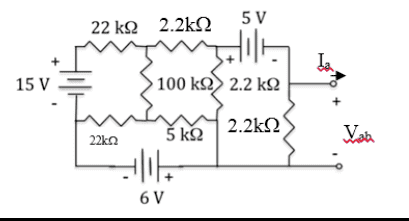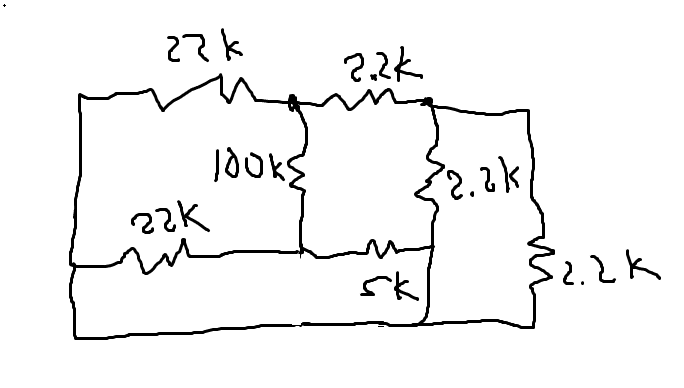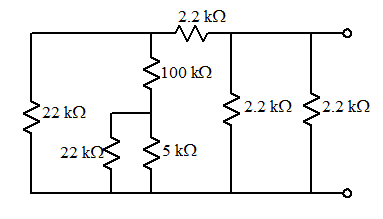# Thevenin Equivalent

## Homework Statement

Find the Thorton equivalent of the circuit shown
[/B]## Homework Equations

Voc=IscRs
Rseries=R1 +R2+.....
Rparallel=R1-1+R2-1+....

## The Attempt at a Solution

So, I thought I should first solve for the Rsource, by shorting all of the voltage sources:However, I dont think the value I am getting is correct.
One value I got is 2111.66 ohms(I combine the 2.2, 2.2, and 5k in series and parallel that with 100k. Then I add the 22k and 22k with the calculated resistance and that is in parallel with the 2.2k)and the other I get is 51663 ohms. (I put the 2.2k from the right with the 2.2k in parallel. Then that combo is in series the other 2.2k and the 5k. That is in parallel with the 100k, and that, finally, is in series with the two 22k)

Please, can someone help me?

## Answers and Replies

gneill
Mentor
I'm not seeing how your 2.2, 2.2, and 5k can be in series.

Try a bit of manipulation of your suppressed-source diagram before you start combining things. Note how the 5K and the 2.2K it connects to are really both tied to the bottom rail? So is one end of both of the 22K resistors. Redraw the circuit to make that more obvious:Does that help?

I think I understand it a little better. Thank you!